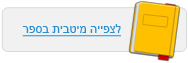עמוד:18 Questions 1 . When is the ball’s potential energy at its maximum ? 2 . When is the ball’s kinetic energy at its maximum ? 3 . What happens to the ball’s kinetic energy when it hits the floor ? 4 . Describe the energy transformations from the moment the ball is released until it returns to its original height ( consider potential energy , kinetic energy , and elastic energy ) . 5 . The following illustration shows the ball in various positions and states over the course of its movement . At the bottom of the illustration is a series of pie diagrams , describing the relative proportions of the different types of energy in each state . For each state , match a corresponding pie diagram ( match numbers to letters ) . Legend : blue – potential energy ; yellow – kinetic energy ; green – elastic energy . Kinetic energy Potential energy Elastic energy© מטח - המרכז לטכנולוגיה חינוכית